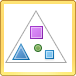# Ternary Class Scatter Plots

 Click the Home | New Graph | Ternary | Ternary Class Scatter Plot command to represent relative percentages in a three-component system. Ternary diagrams are frequently used in chemistry and earth sciences to illustrate rock classification schemes and depict chemical compositions on phase diagrams. A ternary class scatter plot is a ternary plot with symbols for each location based on a required fourth value (Class column). Ternary class plots group data into discrete classes (bins). The data points are displayed using the symbol assigned to the class. Ternary class plots include a legend by default. If you were analyzing the composition of a soil sample, you could use a ternary diagram to illustrate the relative percentages of three minerals found in the sample. The class could be the location, soil type name, or depth of the sample taken. Click the Ternary Class Scatter Plot button to create classed ternary diagrams.To plot data on a ternary class plot, the sum of the three data points (X + Y + Z) in any row must equal 100 percent. If the sum does not equal 100 percent you must normalize your data so that it meets this requirement, otherwise Grapher normalizes the data when it is plotted. Once this relationship has been established between the data points, you need only know the values of any two data points in order to determine the third. Reading a point on a ternary diagrams can be done by looking at the location of each point as it relates to the three axes.

You can add a line connecting the points in a ternary class plot. The line connects the data in the order it appears in the data file. To add a line, open the Line tab in the Property Manager, and then select a line style other than Invisible.Ternary diagrams show percentagesin a three-component system.

## Creating a New Ternary Class Scatter Plot

To create a ternary diagram:

1. Click the Home | New Graph | Ternary | Ternary Class Scatter Plot command.
2. Select a data file in the Open Worksheet dialog. You can select a new data file or you can select an open data file in the Open worksheets section.
3. Click the Open button. A ternary class plot is created using the default properties.

## Editing Ternary Class Scatter Plot Properties

To change the features of a ternary class plot, including the columns used to create the diagram, open the ternary class plot properties by selecting the ternary class plot and editing the properties in the Property Manager. Click the following tabs in the Property Manager to change different properties: